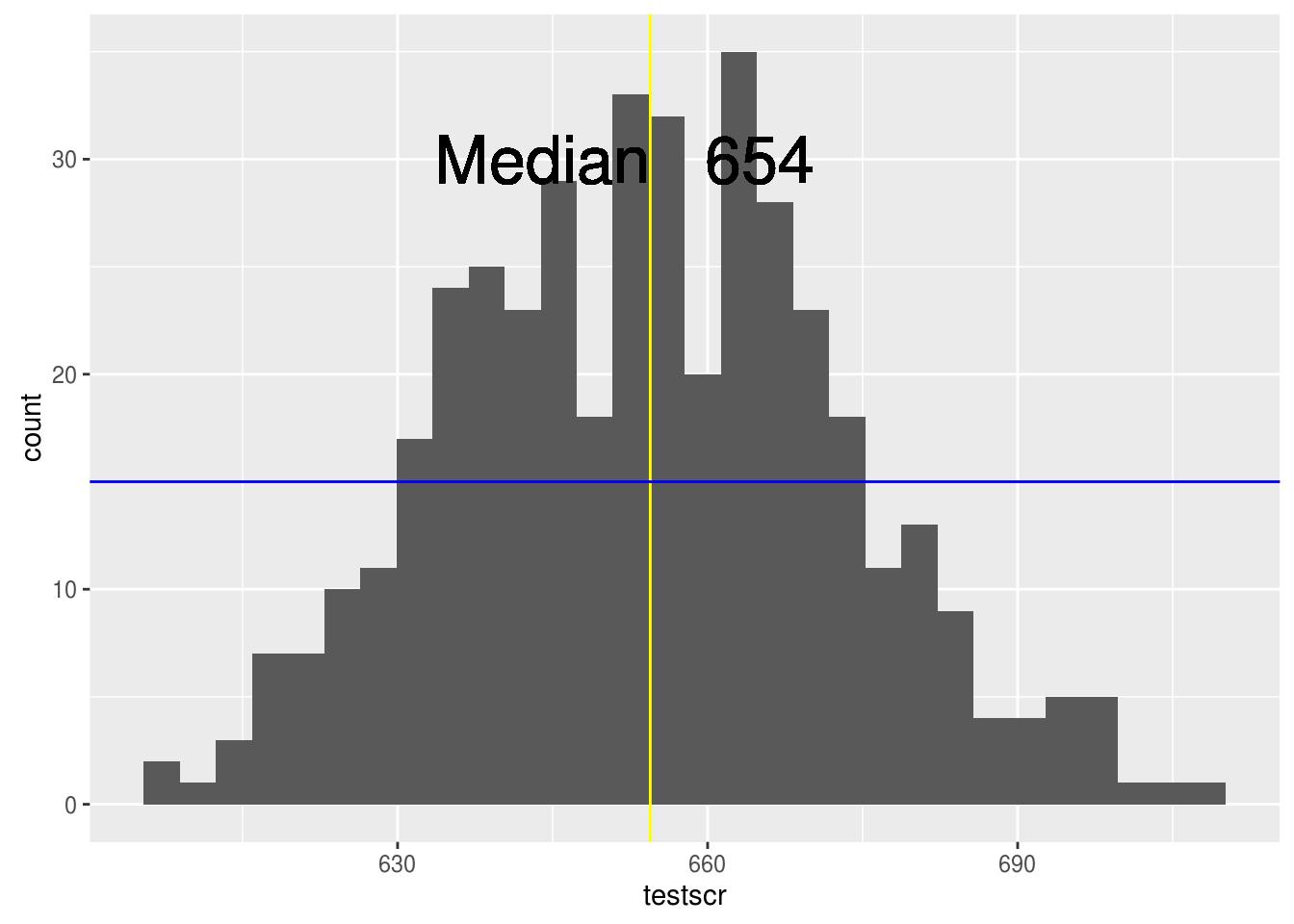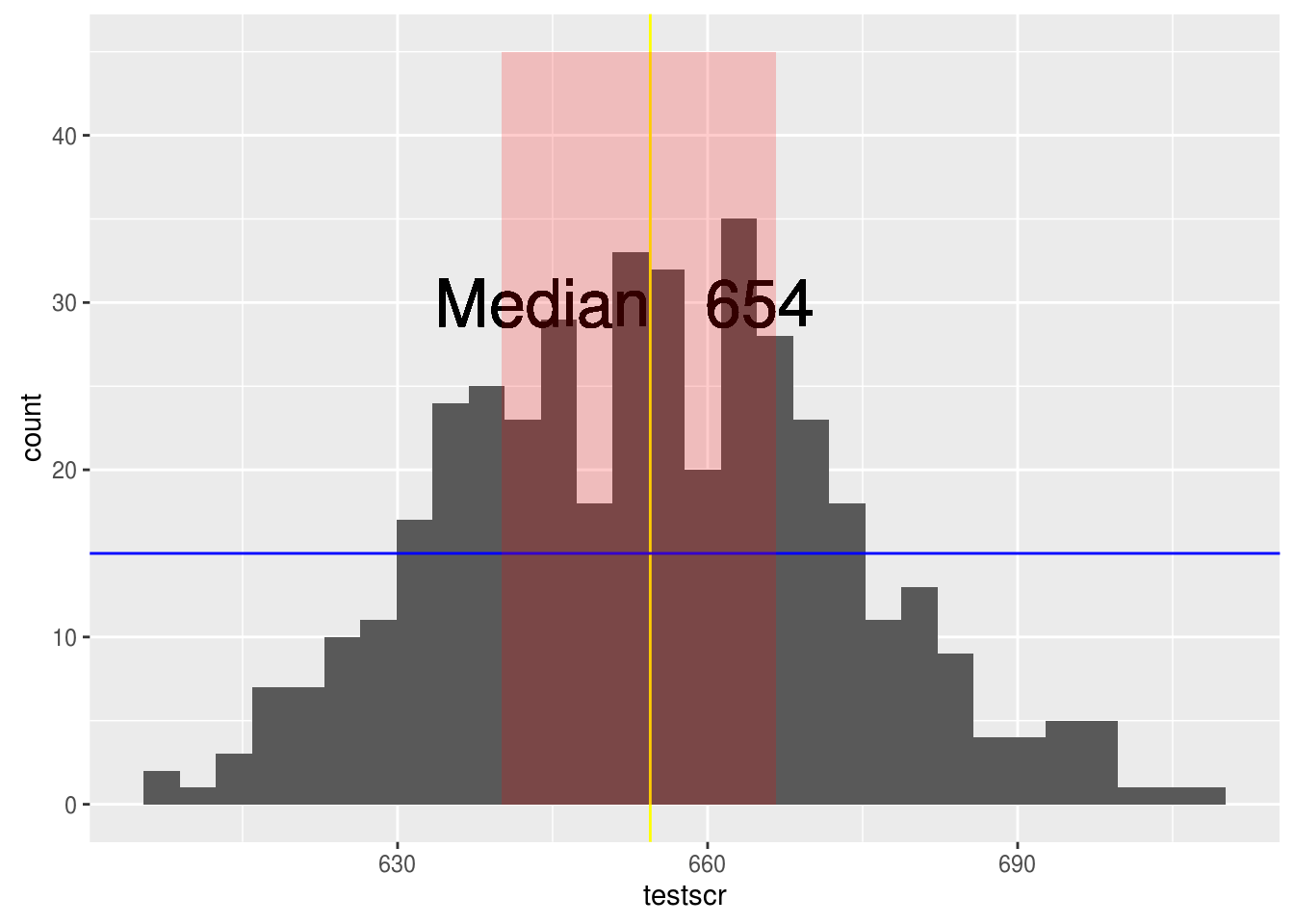# Adding text and Lines to Plots in R

There are times when a researcher may want to add annotated information to a plot. Example of annotation includes text and or different lines to clarify information. In this post we will learn how to add lines and text to a plot. For the lines, we are speaking of lines that are added mainly and not through some sort of statistical transformation such as through regression or smoothing.

In order to do this we will use the “Caschool” data set from the “Ecdata” package and will make several histograms that will display test scores. Below is initial coding information that is needed.

``library(ggplot2);library(Ecdat)``
`data("Caschool")`

There are three lines that can be added manually using ggplot2. They are…

• geom_vline = vertical line
• geom_hline = horizontal line
• geom_abline = slope/intercept line

In the code below, we are going to make a histogram of the test scores in the “Caschool” dataset. We are also going to add a vertical yellow line that is set at where the median is. Below is the code

``````ggplot(Caschool,aes(testscr))+geom_histogram()+
geom_vline(aes(xintercept=median(testscr)),color="yellow")``````By adding aesthetic information to the “geom_vline” function we add the line depicting the median. We will now use the same code but add a horizontal line. Below is the code.

``````ggplot(Caschool,aes(testscr))+geom_histogram()+
geom_vline(aes(xintercept=median(testscr)),color="yellow")+
geom_hline(aes(yintercept=15), color="blue")``````The horizontal line we added was at the arbitrary point of 15 on the y axis. We could have set it anywhere we wanted by specifying a value for the y-intercept.

In the next histogram we are going to add text to the graph. Text provides further explanation about what is happening in the plot. We are going to use the same code as before but we are going to provide additional information about the yellow median line. We are going to explain that the yellow is the median and we will provide the value of the median.

``````ggplot(Caschool,aes(testscr))+geom_histogram()+
geom_vline(aes(xintercept=median(testscr)),color="yellow")+
geom_hline(aes(yintercept=15), color="blue")+
geom_text(aes(x=median(Caschool\$testscr),
y=30),label="Median",hjust=1, size=9)+
geom_text(aes(x=median(Caschool\$testscr),
y=30,label=round(median(testscr),digits=0)),hjust=-0.5, size=9)``````
``

Must of the code above is review but we did add the “geom_text” function. Here is what’s happening. Inside the function we need to add aesthetic information. We indicate that the label =“median” should be placed at the median for the test scores for the x value and at the arbitrary point of 30 for the y-intercept. We also offset the the placement by using the hjust argument.

For the second label we calculate the actual median and have it rounded and have the digits removed. This result is also offset slightly. Lastly, for both text we set the text size to 9 to make it easier to read.

Are next example involves annotating. Using ggplot2 we can actually highlight a specific area of the histogram. In the example below we highlight the middle quartile.

``````ggplot(Caschool,aes(testscr))+geom_histogram()+geom_vline(aes(xintercept=median(testscr)),color="yellow")+
geom_hline(aes(yintercept=15), color="blue")+
geom_text(aes(x=median(Caschool\$testscr),y=30),
label="Median",hjust=1, size=9)+
geom_text(aes(x=median(Caschool\$testscr),y=30,
label=round(median(testscr),digits=0)),hjust=-0.5, size=9)+
annotate("rect",xmin=quantile(Caschool\$testscr, probs = 0.25),
xmax = quantile(Caschool\$testscr, probs=0.75),ymin=0,
ymax=45, alpha=.2, fill="red")``````
``

The information inside the “annotate” function includes the “rect” argument which indicates that the added information is numerical. Next, we indicate that we want the xmin value to be the 25% quartile and the xmax to be the 75% quartile. We also indicate the values for the y axis as well as some transparency with the “alpha” argument as well as the color of the annotated area, which is red.

Are final example involves the use of facets. We are going to split the data by school district type and show how you can add lines to another while not adding lines to a different plot. The second plot will include a line based on median while the first plot will not.

``````ggplot(Caschool,aes(testscr, fill=grspan))+geom_histogram()+
geom_vline(data=subset(Caschool, grspan=="KK-08"),
aes(xintercept=median(testscr)), color="yellow")+
geom_text(data=subset(Caschool, grspan=="KK-08"),
aes(x=median(Caschool\$testscr),y=35), label=round(median
(Caschool\$testscr),
digits=0),
hjust=-0.2,
size=9)+
geom_text(data=subset(Caschool,grspan=="KK-08"),
aes(x=median(Caschool\$testscr), y=35),label="Median",
hjust=1,size=9)+facet_grid(.~grspan)``````
``

Conclusion

Adding lines to text and understanding how to annotate provides additional tools for those who need to communicate data in a visual way.